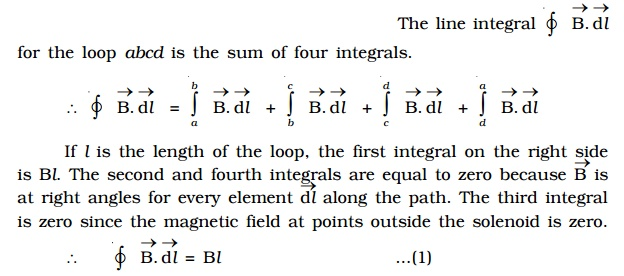Home | | Physics | Magnetic induction due to a long solenoid carrying current

# Magnetic induction due to a long solenoid carrying current

Let us consider an infinitely long solenoid having n turns per unit length carrying a current of I. For such an ideal solenoid (whose length is very large compared to its radius), the magnetic field at points outside the solenoid is zero.

Magnetic induction due to a long solenoid carrying current.

Let us consider an infinitely long solenoid having n turns per unit length carrying a current of I. For such an ideal solenoid (whose length is very large compared to its radius), the magnetic field at points outside the solenoid is zero.A long solenoid appears like a long cylindrical metal sheet (Fig 3.17). The upper view of dots is like a uniform current sheet coming out of the plane of the paper. The lower row of crosses is like a uniform current sheet going into the plane of the paper.

To find the magnetic induction (B) at a point inside the solenoid, let us consider a rectangular Amperean loop abcd.Since the path of integration includes nl turns, the net current enclosed by the closed loop isThe solenoid is commonly used to obtain uniform magnetic field. By inserting a soft iron core inside the solenoid, a large magnetic field is producedwhen a current carrying solenoid is freely suspended, it comes to rest like a suspended bar magnet pointing along north-south. The magnetic polarity of the current carrying solenoid is given by End rule.

End rule

When looked from one end, if the current through the solenoid is along clockwise direction Fig 3.18a, the nearer end corresponds to south pole and the other end is north pole.When looked from one end, if the current through the solenoid is along anti-clock wise direction, the nearer end corresponds to north pole and the other end is south pole (Fig 3.18b)

Study Material, Lecturing Notes, Assignment, Reference, Wiki description explanation, brief detail
Physics : Effects of electric current : Higher Secondary(12 Std) : Magnetic induction due to a long solenoid carrying current |